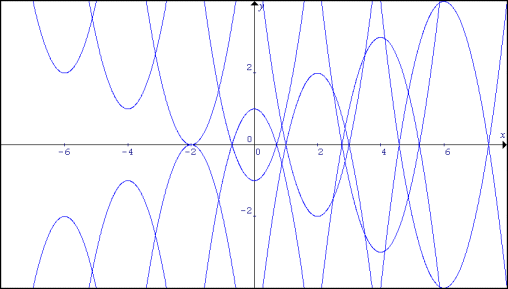#### You may also like### Cubic Spin

Prove that the graph of f(x) = x^3 - 6x^2 +9x +1 has rotational symmetry. Do graphs of all cubics have rotational symmetry?### Sine Problem

In this 'mesh' of sine graphs, one of the graphs is the graph of the sine function. Find the equations of the other graphs to reproduce the pattern.### Parabolic Patterns

The illustration shows the graphs of fifteen functions. Two of them have equations y=x^2 and y=-(x-4)^2. Find the equations of all the other graphs.

# Parabolas Again

##### Age 14 to 18Challenge Level

Here is a pattern for you to experiment with. This one is composed of the graphs of 14 parabolas. You may like to use a graphic calculator or graph drawing software to do this question.

The equations of three of the graphs are: $$y=2(x-6)^2-4$$ $$y=2x^2 -1$$ $$y= -2(x-4)^2 +3$$Find the equations of the other 11 graphs in this pattern. Draw your own pattern and send it to us.